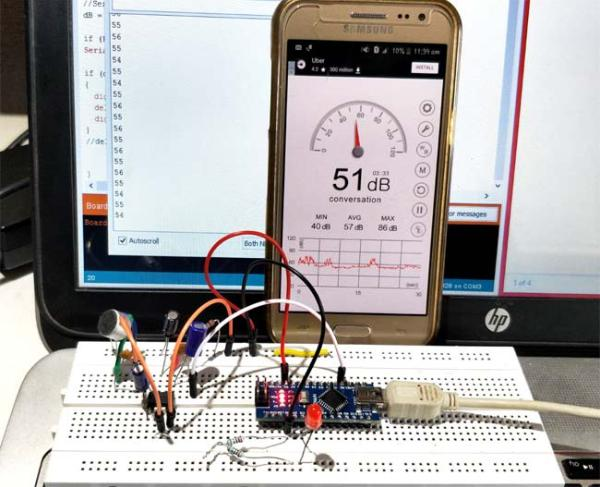# Arduino Voltmeter Prototype

In this instructable, I’ll show you how to make a simple arduino voltmeter. It can measure voltages in excess of 30VDC depending on how you configure it. The smaller the voltage range you want to measure, the more accurate that your meter will be. This project is going to be apart of a gauss meter that I am making to measure magnetic fields strength as well as magnetic field polarity. This instructable should provide a good base for a large number of different applications that will need the arduino to monitor the voltage level of something.

## Step 1: What You Will Need

3. Two resistors. The values depend on the range of voltages you want to measure

## Step 2: Schematic

Wire up your components as shown and choose your resistor values based on the range you want to measure. For example, if you want to measure 0-10V then you could pick R1 as 100k and R2 as 100k. Be sure to measure your resistors before plugging the number into your program to ensure a more accurate measurement.

To calculate your desired voltage range you will need to use the following voltage divider formula.

Vout = (R2*Vin)/(R1+R2)

Vout will need to be 5V for the ADC and Vin will be the max voltage value that you want to measure.

Here are a few voltage range examples:

0-10V : R1=100k, R2=100k

0-15V : R1=100k, R2=50k

0-25V : R1=100k, R2=25k

0-55V : R1=100k, R2=10k

## Step 3: Upload the Code

Before you upload the code, you will need to download the U8glib library from here if you are using an OLED display.

## Step 4: How It Works

This voltmeter uses the arduino’s analog to digital converter on the analog pin and convert 0-5 volts into a value from 0-1023. To measure more than 5 volts, you need use a voltage divider and calculate the voltage in your program. The problem with this is that the arduino’s analog to digital converter still only has a resolution of 1024 points so the higher voltage you want to measure, the less accurate your voltmeter will be.

The ADC on the Arduino is a 10-bit ADC, meaning it only has the ability to detect 1,024 discrete analog levels. For my gauss meter I will probably use a microcontroller with a 16-bit ADC for 65,535 discrete levels and a overall more accurate meter.

Only use this voltmeter to measure positive voltages and never hook it up with the wrong polarity. In my gauss meter instructable, the arduino will measure negative and positive values so wait for that to see how to measure negative values.

#### This Post / Project can also be found using search terms:

• Arduino Voltage Divider 0-25v
• arduino voltmeter projects